## TN State Board 12th Physics Important Questions Chapter 6 Optics

Question 1.
How will you distinguish between a plane, a concave and convex mirror without touching its surface?
We see our own face in each mirror and note the magnification of the image.

• If magnification is more than 1, mirror is plane.
• If magnification is more than 1, mirror is concave.
• If magnification is less than 1, mirror is m convex.

Question 2.
What should be the change in the focal length of n concave mirror when it is held in water?
No change. The focal length of a concave mirror does not depend on the nature of the medium.

Question 3.
What is the cause of refraction of light?
Light travels with different speeds in different media. The bending or refraction of light occurs due to the change in speed of light as it passes from one medium to another.Question 4.
What happens to a focal length of a convex lens, when it is immersed in water?
Power of a lens, P ∝ (p – 1)
As wµg < aµg, so power of the glass lens will decrease when it is immersed in water.

Question 5.
Why is the smote of cigarette blue?
This is because smoke particle scatter blue light preferentially.

Question 6.
Why do we observe a spectrum in the .minimum deviation position?
In the position of minimum deviation, the rays of different colours will suffer almost the same deviation and they can be focussed on the same screen.

Question 7.
A right angle prism is placed before an object sn the rwo positions shown in figure. The prism is made of crown glass with critical angle equal to 41°. Trace the paths of two rays from P and Q. normal to the hypotenuse in figure (a) and parallel to the hypotenuse in figure (b).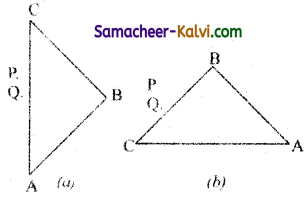As the angle of incidence inside the prism is greater than the critical angle (41°), the light rays suffer total internal reflection twice in case (a) and once in case (b). In both cases, inverted image P’ Q’ is formed.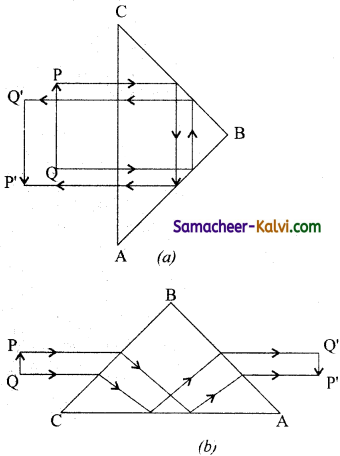Question 8.
A light wave enters from air into glass. How will the following be affected, (i) Energy of t he wave, (ii) Frequency of the wave.
(i) Energy of the wave decreases because a part of the light wave is reflected back into air.
(ii) Frequency of the wave remains unchanged.

Question 9.
What are the reasons to believe that light is a wave motion?
Light undergoes interference, diffraction and polarisation. These phenomena establish that light is a wave motion.

Question 10.
Why are coherent sources necessary to produce a sustained interference pattern?
Coherent sources have a constant phase difference. This ensures that the positions of maxima and minima do not change with time. i.e., a sustained interference pattern is obtained.Question 11.
Two slits in young’s double slit experiment are illuminated by two different lamps emitting light of the same wavelength. Will you observe the interference pattern ? Justify your answer.
No. The light wave emitted by two different lamps cannot be coherent. So the conditions of maxima and minima of intensity will change rapidly on the screen, producing uniform illumination.

Question 12.
Why is interference pattern not detected, when the two coherent sources are far apart?
When the distance d between the two coherent sources is large, the fringe width (β ∝ $$\frac{1}{d}$$) a becomes too small to be detected. The interference pattern cannot be observed.

Question 13.
State the essential condition for diffraction of light to occur.
Diffraction of light occurs when size of the obstacle or the aperture is comparable to the wavelength of light.

Question 14.
Will ultrasonic waves show any polarisation ? Give reasons for your answer.
No, ultrasonic waves are longitudinal in nature. So they cannot be polarised.Question 15.
Name three properties, which are mutually perpendicular to each gives in a plane, polarised light wave.
Plane of vibration, plane of polarisation and direction of propagation of the light wave.

Question 16.
A disc is placed on a surface of pond which has refractive index 5/3. A source of light is placed 4 cm below the surface of liquid. What is the minimum diameter of the disc needed to that light is not coming out. The light rays will be totally internally reflected if i > ic.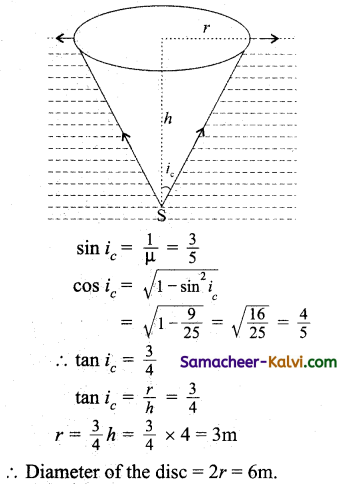Question 17.
In young’s double slit experiment, the intensity ratio of two coherent sources are 49 :1 Calculate the maximum and minimum intensities.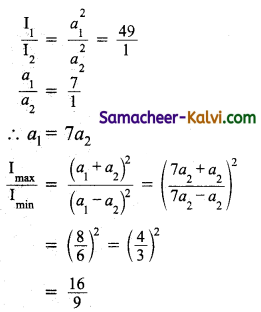Ratio of maximum intensity and minimum intensity = 16 : 9

Question 18.
A concave mirror has a focal length of 18 cm. Rnd the position or positions of an object forwhich the image is double of the object size.
Let the distance of object from mirror is x.
By sign conventions,
f = – 18 cm
u = – x
If image is real, m = -2
Magnification, m = $$\frac{-v}{u}$$
-2 = $$\frac{-v}{u}$$
v = – 2x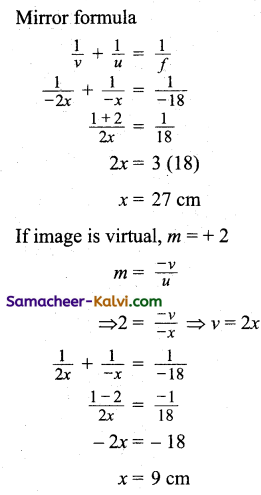The distance of the object from the mirror are 9 cm or 27 cm.Question 19.
A ray falls on a prism ABC (AB = BC) and travels as shown in figure. What is the minimum refractive index of the prism material.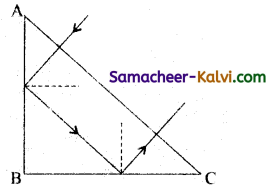Since AB = BC
∠BAC = ∠ACB = 45°
Total internal reflection takes place at AB and BC.
i.e., 45° ≥ C
sin 45° ≥ sin C
$$\frac{1}{\sqrt{2}}$$ ≥ $$\frac{1}{\mu}$$
µ ≥ √2
∴ µmin = √2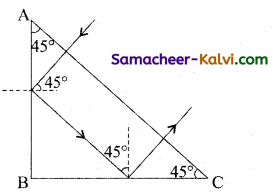Question 20.
The refractive index of glass is 1.5 and that of water is 13. If the speed of light in water is 2.25 × 108 ms-1, what is the speed of light in glass?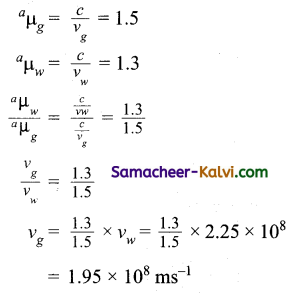Question 21.
A light of wavelength 6000 Å in air, enters a medium with refractive index 1.5. What will be the frequency and wavelength of light in that medium.
When light travels from air to the refracting medium, in frequency remains unchanged.
∴ υ = $$\frac{c}{\lambda}=\frac{3 \times 10^{8}}{6000 \times 10^{-10}}$$
= 5 × 1014 Hz
wavelength of light in the medium
λ’ = $$\frac{\lambda}{\mu}=\frac{6000 \times 10^{-10}}{1.5}$$ = 4000 ÅQuestion 22.
The near point and far point of a person are 40 cm and 250 cm respectively. Determine the power of the lens he / she should use while reading a book kept at the distance 25 cm from the eye.
focal length f = $$\frac{y \mathrm{D}}{y-\mathrm{D}}$$

= $$\frac{40 \times 25}{40-25}=\frac{200}{3}$$cm = $$\frac{2}{3}$$ cm

Power of the lens P = $$\frac{1}{f}$$
= $$\frac{3}{2}$$ D = 1.5 D.

Question 23.
The focal length of the objective and eye lenses of a microscope are 1.6 cm and 2.5 cm respectively. The distance between the two lenses is 21.7 cm. If the final image is formed at infinity, what is the linear magnification?
For the objective lens,
υ0 = L – fe
= 21.7 – 2.5 = + 19.2 cm
Here f0 = + 1.6 cm
$$\frac{1}{u_{0}}=\frac{1}{v_{0}}-\frac{1}{f_{0}}$$

$$\frac{1}{u_{0}}=\frac{1}{19.2}-\frac{1}{1.6}=\frac{17.6}{19.2 \times 1.6}$$

Magnification of the microscope, the image at infinity m = $$\frac{v_{0}}{u_{0}} \cdot \frac{\mathrm{D}}{f_{e}}$$

= $$\frac{17.6}{19.2 \times 1.6} \times 19.2 \times \frac{25}{2.5}$$ = 11 × 10 = 110

Question 24.
A thin convex lens of refractive index 1.5 has 20 cm focal length in air. If the lens is completely immersed in a liquid of refractive index 1.6, find its focal length.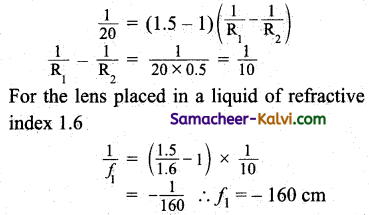Question 25.
A slit of width ‘a’ is illuminated by red light of wavelength 6500 A. For what value of ‘a’ will
(i) the first minimum fall at an angle of diffraction of 30 and
(ii) The first minimum fall at an angle of diffraction of 30°.
(i) For first minimum of diffraction pattern a sin θ = λ
a = $$\frac{\lambda}{\sin \theta}=\frac{6500 \times 10^{-10}}{0.5}$$
= 1.3 × 10-6 m.

(ii) For first secondary maximum diffraction pattern, a sin θ = $$\frac{3 \lambda}{2}$$
a = $$\frac{3 \lambda}{2 \sin \theta}=\frac{3 \times 6500 \times 10^{-10}}{2 \times 0.5}$$
= 1.95 × 10-6 m.Question 26.
For what distance is ray optics a good approximation when the aperture is 3mm wide and the wavelength is 500 nm?
Here a = 3mm = 3× 10-3
λ = 500 nm = 500 × 10-9 m.
Fresnel distance DF = $$\frac{a^{2}}{\lambda}$$
= $$\frac{\left(3 \times 10^{-3}\right)^{2}}{500 \times 10^{-9}}$$ = 18 m

Question 27.
An object is placed at a distance of 30.0 cm from a concave mirror of focal length 20.0 cm. What Is the nature of the image and image distance from the mirror.
Here, u = – 30 cm, f = – 20 cm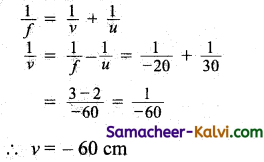The image is formed at a distance 60 cm to the left of the concave mirror.
Magnification m = $$-\frac{v}{u}=-\frac{(-60)}{(-30)}$$ = – 2
As the sign of magnification is negative, the image is inverted, magnified by two times and real image.Multiple Choice Questions:

Question 1.
Light wave:
(a) require air to travel
(b) can travel in perfect vacuum
(c) require an electric field to travel
(d) require an magnetic field to travel
(b) can travel in perfect vacuum

Question 2.
If the wavelength of incident light is increased by four times then the amount of scattering will be:
(a) increased by 256 times
(b) decreased by 256 times
(c) increased by 16 times
(d) decreased by 64 times
(b) decreased by 256 times

Question 3.
Amount of scattering of light is inversely proportional to the fourth power of the wavelength is given by:
(a) Wien’s law
(b) Rayleigh’s law
(c) Stefan’s law
(d) Shell’s law
(b) Rayleigh’s lawQuestion 4.
Among the spectrum of visible light, shortest wavelength is ________ colour.
(a) violet
(b) red
(c) yellow
(d) blue
(a) violet

Question 5.
If the source is at infinity, the shape of the wavefront is:
(a) spherical
(b) cylindrical
(c) plane
(d) elliptical
(c) plane

Question 6.
When the distance between the source and the screen is increased in young’s double slit experiment, the fringe:
(a) width increases
(b) width decreases
(c) disappears
(d) width remains constant
(a) width increasesQuestion 7.
The bending of light rays at any edge is known as:
(a) interference
(b) polarisation
(c) refraction
(d) diffraction
(d) diffraction

Question 8.
The grating element is:
(a) number of lines per metre
(b) width of grating
(c) combined width of a slit and ruling
(d) area of the grating
(c) combined width of a slit and ruling

Question 9.
Plane polarised light can be produced by:
(a) telescope
(b) microscope
(c) spectrometer
(d) pile of plates
(d) pile of platesQuestion 10.
Polarisation of light by reflection was discovered by: .
(a) Fraunhofer
(b) Fresnel
(c) Malus
(d) Bartholinus
(c) Malus

Question 11.
Which one of the following waves cannot be polarised?
(a) x-rays
(b) Tranverse wave in string
(c) Longitudinal waves in gas
(c) Longitudinal waves in gas

Question 12.
The face angles of a Nicol prism is:
(a) 60° and 118°
(b) 60° and 120°
(c) 130° and 50°
(d) 72° and 108°
(d) 72° and 108°

Question 13.
An example of uniaxial crystal is:
(a) mica
(b) quartz
(c) totaz
(d) selenite
(b) quartzQuestion 14.
An example of biaxial crystal is:
(a) calcite
(b) quartz
(c) mica
(d) tourmaline
(c) mica

Question 15.
According to Brewster’s law:
(a) µ = tan ip
(b) ip = tan µ
(c) tan ip = $$\frac{1}{\mu}$$
(d) tan ip = µ – ip
(a) µ = tan ip

Question 16.
In a pile of plates, the angle of inclination of the plates to the axis of the tube is:
(a) 90°
(b) 51.5°
(c) 32.5°
(d) 33.7°
(c) 32.5°Question 17.
The angle of polarisation for glass is:
(a) 52.5°
(b) 37.5°
(c) 32.5°
(d) 56.3°
(b) 37.5°

Question 18.
The refractive index for ordinary light for sodium light is:
(a) 1.5
(b) 1.55
(c) 1.658
(d) 1.486
(c) 1.658

Question 19.
The refractive index for Canada balsam is:
(a) 1.486
(b) 1.523
(c) 1.5
(d) 1.628
(b) 1.523

Question 20.
The amount of scattering is directly proportional to:
(a) $$\frac{1}{\lambda^{4}}$$
(b) λ4
(c) $$\frac{1}{\lambda^{-4}}$$
(d) λ-4
(d) λ-4Question 21.
A point object is placed at a distance of 20 cm from a convex mirror of focal length 20 cm. The image will form at:
(a) infinity
(b) focus
(c) pole
(d) 10 cm behind the mirror
(d) 10 cm behind the mirror

Question 22.
A fish at a. depth of 20 cm in water is viewed by an observer on the tank of a lake. To what height the image of the fish is raised:
(a) 5 cm
(b) 12 cm
(c)20cm
(d) 10 cm
(a) 5 cm

Question 23.
A fish is a little away below the surface of a lake. If the critical angle is 49°, then the fish could see things above the wave surface with in an angular of 0° where 0 is:
(a) 49°
(b) 90°
(c) 98°
(d) 24°
(c) 98°

Question 24.
If I1 and I2 be the size of the images respectively for the two positions of lens in the displacement method, then the size of the object is:
(a) $$\sqrt{I_{1} I_{2}}$$
(b) I1/I2
(c) I1 I2
(d) $$\sqrt{\frac{\mathrm{I}_{1}}{\mathrm{I}_{2}}}$$
(a) $$\sqrt{I_{1} I_{2}}$$Question 25.
The plane surface of a plane – convex lens of focal length/is silvered. It will behave as:
(a) plane mirror
(b) concave mirror of focal length $$\frac{f}{2}$$
(c) convex mirror of focal length 2f
(d) none of the above
(b) concave mirror of focal length $$\frac{f}{2}$$

Question 26.
A lens of power – 2 dioptres is placed in contact with a lens of power + 1 dioptre. The combination will behave like:
(a) A convergent lens of focal length 100 cm
(b) A divergent lens of focal length 100 cm
(c) A divergent lens of focal length 50 cm
(d) A convergent lens of focal length 50 cm
(b) A divergent lens of focal length 100 cm

Question 27.
An object 4 cm tall is placed 90 cm from a concave spherical mirror which as a radius of curvature of 20 cm. The size of the image is:
(a) 0.11 cm
(b) 0.55 cm
(c) 0.6 cm
(d) 0.50 cm
(d) 0.50 cm

Question 28.
The focal length of concave mirror is 50 cm. Where an object be placed so that its image is two times and inverted:
(a) 75 cm
(b) 10cm
(c) 68 cm
(d) 50 cm
(a) 75 cmQuestion 29.
The minimum distance between an object and its real image formed by convex lens is:
(a) 1.5 f
(b) 2.5 f
(c) 4 f
(d) 2 f
(c) 4 f

Question 30.
The angle of minimum deviation for a prism is 40° and the angle of the prism is 60°. The angle of incidence in this prism will be:
(a) 50°
(b) 100°
(c) 60°
(d) 30°
(a) 50°

Question 31.
If mx and m2 be the linear magnification of the objective lens and eye lens of a compound microscope, then the magnifying power of the compound microscope will be:
(a) m1 – m2
(b) $$\sqrt{m_{1}+m_{2}}$$
(c) $$\frac{m_{1}+m_{2}}{2}$$
(d) m1 × m2
(d) m1 × m2

Question 32.
The magnification produced by the objective lens and the eye lens of compound microscope are 20 and 5 respectively. The magnifying power of this microscope is:
(a) 25
(b) 15
(c) 40
(d) 100
(d) 100Question 33.
When a ray of light enters a glass slab from air:
(a) in wavelength decreases
(b) in wavelength increases
(c) its frequency increases
(d) neither in wavelength nor in frequency changes.
(a) in wavelength decreases

Question 34.
A ray of light passes through four transparent media with refractive indices μ1, μ2, μ3 and μ4 as shown in fig.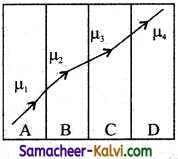The surfaces of all media are parallel. If the emerged ray CD is parallel to the incident ray AB, we must have:

(a) μ1 = μ2
(b) μ2 = μ3
(c) μ3 = μ4
(d) μ4 = μ1
(d) μ4 = μ1

Question 35.
A point object is placed at the centre of a glass sphere of radius 6 cm and refractive index 1.5. The distance of the virtual image from the surface of the sphere is:
(a) 2 cm
(b) 4 cm
(c) 6 cm
(d) 112 cm
(c) 6 cm

Question 36.
An equilateral prism is placed on a horizontal surface. A ray PQuestion is incident onto it. For minimum deviation: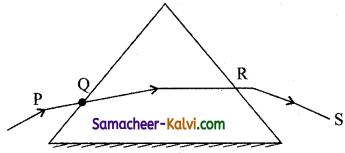(a) PQ is horizontal
(b) QR is horizontal
(c) RS is horizontal
(d) any one will be horizontal
(b) QR is horizontalQuestion 37.
In a compound microscope, the intermediate image is:
(a) virtual, erect and magnified
(b) real, erect and magnified
(c) real, inverted and magnified
(d) virtual, erect and reduced
(c) real, inverted and magnified

Question 38.
A converging lens is used to form an image on a screen. When the upper half of the lens is covered by a opaque screen:
(a) half the image will disappear
(b) complete image will disappear
(c) intensity of image will decrease
(d) intensity of image will increase
(c) intensity of image will decrease

Question 39.
To get three images of a single object, one should have two plane mirrors at an angle of:
(a) 60°
(b) 90°
(c) 120°
(d) 30°
(b) 90°

Question 40.
Which of the following is used in optical fibres?
(a) Total internal reflection
(b) Scattering
(c) Diffraction
(d) Refraction
(a) Total internal reflectionQuestion 41.
An astronomical telescope has a large aperture to:
(a) reduce spherical aberration
(b) have high resolution
(c) increase span of observation
(d) have low dispersion
(b) have high resolution

Question 42.
A boy 1.5 m tall with his eye level at 1.35 m stands before a mirror fixed on a wall. The minimum length of mirror required to view the complete image of boy is:
(a) 0.7 m
(b) 0.5 m
(c) 0.75 m
(d) 0.85 m
(c) 0.75 m

Question 43.
A pencil of light rays falls on a plane mirror and forms a real image, so the incident rays are:
(a) parallel
(b) diverging
(c) converging
(d) statement is false
(c) converging

Question 44.
For a real object, which of the following can produce a real image?
(a) concave mirror
(b) plane mirror
(c) convex mirror
(d) concave lens
(a) concave mirrorQuestion 45.
Which mirror is to be used to obtain a parallel beam of light from a small lamp?
(a) plane mirror
(b) convex mirror
(c) concave mirror
(d) none of the above
(c) concave mirror

Question 46.
The reddish appearance of rising and setting sun is due to:
(a) reflection of light
(b) diffraction of light
(c) scattering of light
(d) interference of light
(c) scattering of light

Question 47.
For normal vision, the distance of distinct vision of eye is:
(a) 30 cm
(b) 25 cm
(c) 40 cm
(d) infinite
(b) 25 cm

Question 48.
In the formation of a rainbow, the light from the sun on water droplets undergoes:
(a) dispersion only
(b) only total internal reflection
(c) dispersion and total internal reflection
(d) none of the above
(c) dispersion and total internal reflectionQuestion 49.
In order to increase the angular magnification of a simple microscope, one should increase:
(a) the object size
(b) the aperture of the lens
(c) the focal length of the lens
(d) the power of the lens
(d) the power of the lens

Question 50.
F1 and F2 are focal lengths of objective and eyepiece respectively of the telescope. The angular tnagnification fof the given telescope is equal to:
(a) $$\frac{\mathrm{F}_{1}}{\mathrm{~F}_{2}}$$

(b) $$\frac{\mathrm{F}_{2}}{\mathrm{~F}_{1}}$$

(c) $$\frac{\mathrm{F}_{1} \mathrm{~F}_{2}}{\mathrm{~F}_{1}+\mathrm{F}_{2}}$$

(d) $$\frac{\mathrm{F}_{1}+\mathrm{F}_{2}}{\mathrm{~F}_{1} \mathrm{~F}_{2}}$$

(a) $$\frac{\mathrm{F}_{1}}{\mathrm{~F}_{2}}$$

Question 51.
Focal length of objective and eyepiece of telescope are 200 cm and 10 cm respectively. What is the length of telescope for normal adjustment?
(a) 190 cm
(b) 250 cm
(c) 225 cm
(d) 210 cm
(d) 210 cm

Question 52.
The focal length of the objective and eye piece of a telescope are respectively 100 cm and 2 cm. The moon subtends angle of 0.5°, the angle subtended by the moon’s image will be:
(a) 10°
(b) 25°
(c) 100°
(d) 75°
(b) 25°Question 53.
Two mirrors are kept at 60° to each other and a body is placed at middle. The total number of images formed is:
(a) six
(b) four
(c) five
(d) three
(c) five

Question 54.
Apoint source kept at a distance of 1000 m has a illumination I. To change the illumination to 161, the new distance should become:
(a) 250 m
(b) 500 m
(c) 750 m
(d) 800 m
(a) 250 m

Question 55.
What should be the maximum acceptable angles at the air-core interface on an optical fibre if nx and n2 are the refractive indices of the core and cladding respectively?
(a) sin-1 $$\left(\frac{n_{1}}{n_{2}}\right)$$

(b) sin-1 $$\sqrt{n_{1}^{2}-n_{2}^{2}}$$

(c) tan-1 $$\frac{n_{2}}{n_{1}}$$

(d) sin-1 $$\left(\frac{n_{2}}{n_{1}}\right)$$

(b) sin-1 $$\sqrt{n_{1}^{2}-n_{2}^{2}}$$

Question 56.
An object is immersed in a fluid. In order that the object becomes invisible, it should:
(a) behave as a perfect reflector
(b) absorb all light falling on it
(c) have refractive index one
(d) have refractive index exactly matching with that of the surrounding fluid.
(d) have refractive index exactly matching with that of the surrounding fluid.Question 57.
Mirage is a phenomenon due to:
(a) reflection of light
(b) refraction of light
(c) total internal reflection of light
(d) diffraction of light
(c) total internal reflection of light

Question 58.
One can not see through fog, because:
(a) fog absorbs the light
(b) light suffers total reflection at droplets
(c) refractive index of the fog is infinity
(d) light is scattered by the droplets
(d) light is scattered by the droplets

Question 59.
Ray optics is valid, when characteristic dimensions are:
(a) much smaller than the wavelength of light
(b) much larger than the wavelength of light
(c) of the source order as the wavelength
(d) of the order of one millimetre
(b) much larger than the wavelength of light

Question 60.
If the focal length of objective lens is increased, then magnifying power of:
(a) microscope will increase but that of telescope decrease
(b) microscope and telescope both will increase
(c) microscope and telescope both will decrease
(d) microscope will decrease but that of the telescope will increase
(d) microscope will decrease but that of the telescope will increaseQuestion 61.
Mark the correct option.
(a) If the incident rays are converging, we have a real object.
(b) If the final rays are converging, we have a real image.
(c) The image of a virtual object is called a virtual image.
(d) If the image is virtual, the corresponding object is called a virtual object.
(b) If the final rays are converging, we have a real image.

Question 62.
Which of the following is not due to total internal reflection?
(a) Brilliance of diamond
(b) Working of optical fibre
(c) Difference between apparent and real depth of a pond.
(d) Mirage on hot summer days.
(c) Difference between apparent and real depth of a pond.

Question 63.
Pick out the correct statements about optical fibres from the following:
(i) Optical fibres are used for the transmission and receiving electrical signals only.
(ii) Optical fibres are used for transmitting and receiving electrical signals.
(iii) The intensity of light signals sent through optical fibres suffer very small loss.
(iv) Optical fibres effectively employ the principle of multiple total internal reflections.
(v) Optical fibres are glass fibres coated with a thin layer of a material with lower refractive index.

(a) (i) and (ii)
(b) (ii) and (iii)
(c) (iii) and (iv)
(d) (ii), (iii), (iv) and (v)
(d) (ii), (iii), (iv) and (v)

Question 64.
Consider telecommunication through optical fibres, which of the following statements is not true?
(a) optical fibres may have homogeneous core with a suitable cladding.
(b) Optical fibres can be of graded refractive index.
(c) Optical fibres are subj ect to electromagnetic interference from outside.
(d) Optical fibres have extremely low transmission loss.
(c) Optical fibres are subj ect to electromagnetic interference from outside.Question 65.
Choose the correct option regarding lenses in contact.
(a) the equivalent focal length is $$\frac{1}{f}=\frac{1}{f_{1}}+\frac{1}{f_{2}} a a a \frac{1}{f_{2}}$$

(b) when two lenses are placed at a separation d, if object is at ∞, the two lenses can be replaced by a single lens of focal length $$\frac{1}{f}=\frac{1}{f_{1}}+\frac{1}{f_{2}}-\frac{d}{f_{1} f_{2}}$$

(c) The equivalent lens is to be placed at a distance $$\frac{d f}{f_{1}}$$ behind second lens.

(d) all options are correct.
(d) all options are correct.

Question 66.
Which of the following is correct regarding telescope?
(a) In Terrestrial telescope, three convex lenses are used and final image is erect.
(b) In Astronomical telescope, aperture and focal length of objective is larger than eyepiece.
(c) In Galilean telescope, two lenses, one convex (objective) and one concave (eye piece) are used.
(d) All options are correct
(d) All options are correct

Question 67.
When we see an object, the image formed on the retina is:
(i) real
(ii) virtual
(in) 25 cm
(iv) 1 m

(a) (i) and (ii)
(b) (ii) and (iii)
(c) (ii) and (iv)
(d) (i) and (iv)
(d) (i) and (iv)

Question 68.
Match the elements of Table-I and Table-II.

 Table – 1 Table  – II (i) Myopia (A) Bifocal lens (ii) Hypermetropia (B) Cylindrical lens (iii) Presbyopia (C) Concave lens (iv) Astigmatism (D) Convex lens

(a) (i) – (C); (ii) – (D); (iii) – (A); (iv) – (B);
(b) (i) – (D); (ii) – (C); (iii) – (A); (iv) – (B);
(c) (i) – (A); (ii) – (B); (iii) – (C); (iv) – (D);
(d) (i) – (B); (ii) – (D); (iii) – (A); (iv) – (C);
(b) (i) – (D); (ii) – (C); (iii) – (A); (iv) – (B);Question 69.
Match the elements of Table-I and Table-II.

 Table I Table  II (i) When magnification is positive (A) real image (ii) When magnification is negative (B) magnified image (iii) If | m | > 1 (C) diminished image (iv) If | m | < 1 (D) virtual image

(a) (i) – (D); (ii) – (A); (iii) – (B); (iv) – (C);
(b) (i) – (B); (ii) – (C); (iii) – (D); (iv) – (A);
(c) (i) – (C); (ii) – (B); (iii) – (A); (iv) – (B);
(d) (i) – (C); (ii) – (D); (iii) – (D); (iv) – (A);
(a) (i) – (D); (ii) – (A); (iii) – (B); (iv) – (C);

Question 70.
A green light is incident from the Water to the air-water interface at the critical angle (θ). Select the correct statement.
(a) The entire spectrum of visible light will come out of the water at an angle of 90° to the normal.
(b) The spectrum of visible is less than that of green light will come out to the air medium.
(c) The spectrum of visible light whose frequency is more than that of green light will come out to the air medium.
(d) The entire spectrum of visible light will come out of the water at various angles to the normal.
(b) The spectrum of visible is less than that of green light will come out to the air medium.Assertions and Reasons:

In each of the following questions, a statement of assertion (A) is given followed by a corresponding statement of reason (R) just below it. Of the statements, mark the correct answer is:
(a) If both assertion and reason are true and reason is the correct explanation of assertion.
(b) If both assertion and reason are true but reason is not the correct explanation of assertion.
(c) If assertion is true but reason is false.
(d) If both assertion and reason are false.

Question 71.
Assertion:
A total reflecting prism is used to erect the inverted image without deviation.
Reason:
Rays of light incident parallel to base of prism emerge out as parallel rays.
(a) If both assertion and reason are true and reason is the correct explanation of assertion.
Hint:
The emergent beam is parallel to the incident beam. The rays do not suffer any deviation, only their order is reversed. So a total reflecting prism can be used as an erecting prism.

Question 72.
Assertion:
If objective and eye lenses of a microscope are interchanged, then it can work as telescope.
Reason:
The objective lens of telescope has small focal length.
(d) If both assertion and reason are false.
Hint:
A microscope cannot work as telescope by interchanging its two lenses. These two lenses have short focal length and so the difference fe – f0 is small. On the otherhand, in a telescope, the objective has much longer focal length than the eyepiece.Question 73.
Assertion:
The relation among u, v and / for the spherical mirror is valid for mirrors whose sizes are very small compared to their radii of curvature.
Reason:
The laws of reflection are strictly valid for plane surfaces but not for large spherical surfaces.
(c) If assertion is true but reason is false.
Hint:
The laws of reflection are valid for plane as well as curved surfaces.

Question 74.
Assertion:
A red object appears dark in the yellow light.
Reason:
The red colour is scattered less.
(b) If both assertion and reason are true but reason is not the correct explanation of assertion.
Hint:
A red object appears dark in the yellow light because it absorbs yellow light incident on it.

Question 75.
Assertion:
The clouds in sky generally appears to the whitish.
Reason:
Scattering due to clouds is efficient in equal measure at all wavelengths.
(a) If both assertion and reason are true and reason is the correct explanation of assertion.Question 76.
Assertion:
Endoscopy involves use of optical fibres to study internal organs.
Reason:
Optical fibres are based on phenomenon of total internal reflection.
(a) If both assertion and reason are true and reason is the correct explanation of assertion.

Question 77.
Assertion:
It is not possible to have interference between the waves produced by two violins.
Reason:
For interference of two waves the phase difference between the waves must remain constant.
(a) If both assertion and reason are true and reason is the correct explanation of assertion.
Hint:
The waves produced by the two violins are not coherent.

Question 78.
Assertion:
The pattern and position of fringes always remain same even after the introduction of transparent medium in a path of one of the slits.
Reason:
The central fringe is bright or dark depends upon the initial phase difference between the two coherent sources.
(d) If both assertion and reason are false.
Hint:
The transparent plate introduces the path difference (p -1) t between the two waves and hence changes the position of the central fringe.

Question 79.
Assertion:
To increase resolving power of a telescope, the aperture (a) of the objective should be large.
Reason:
The resolving power of the telescope 1Sg,Venby 1.22X-‘
(a) If both assertion and reason are true and reason is the correct explanation of assertion.Question 80.
Assertion:
Static crashes are heard on radio, when lightening flash occurs in the sky.
Reason: